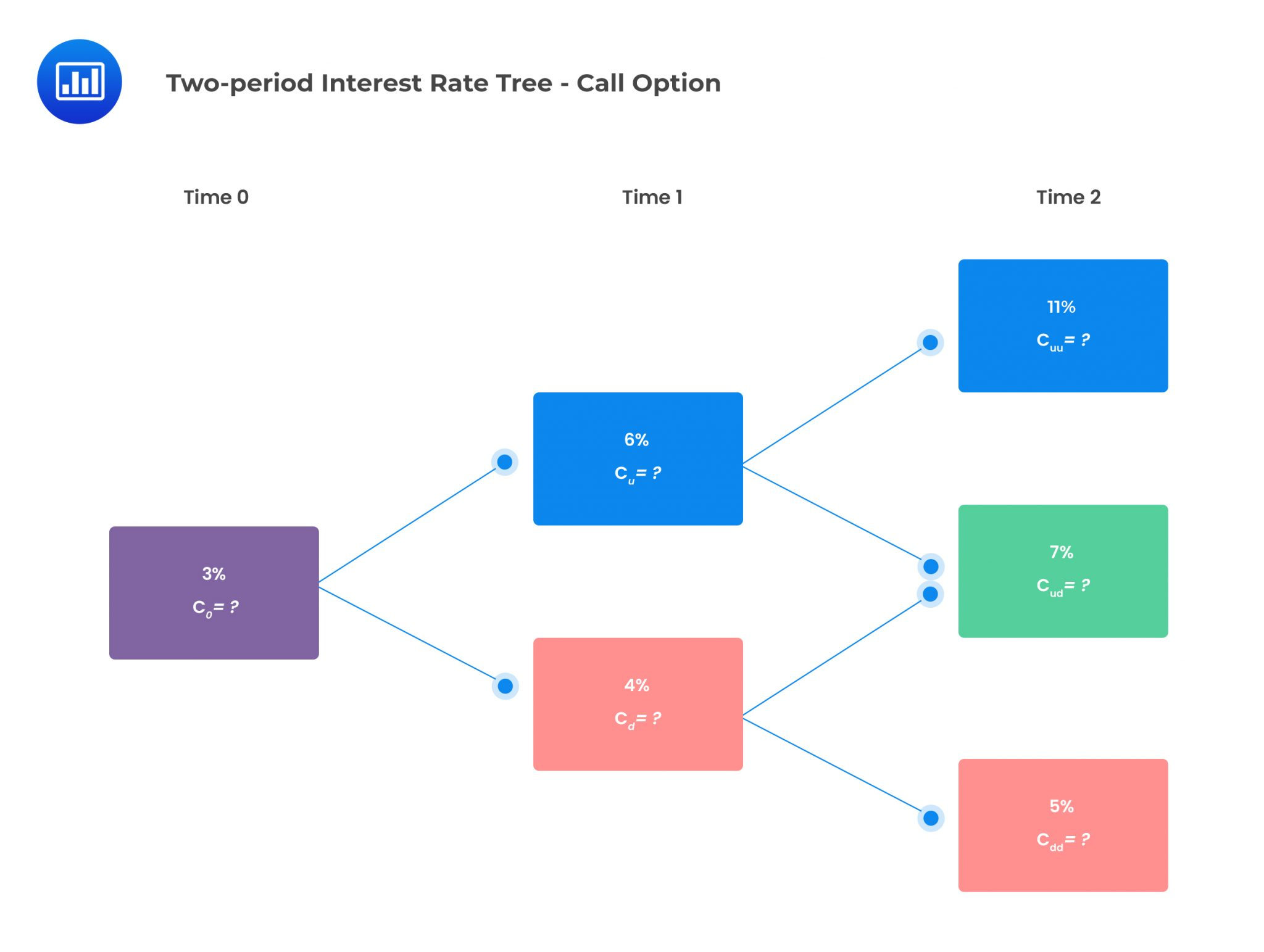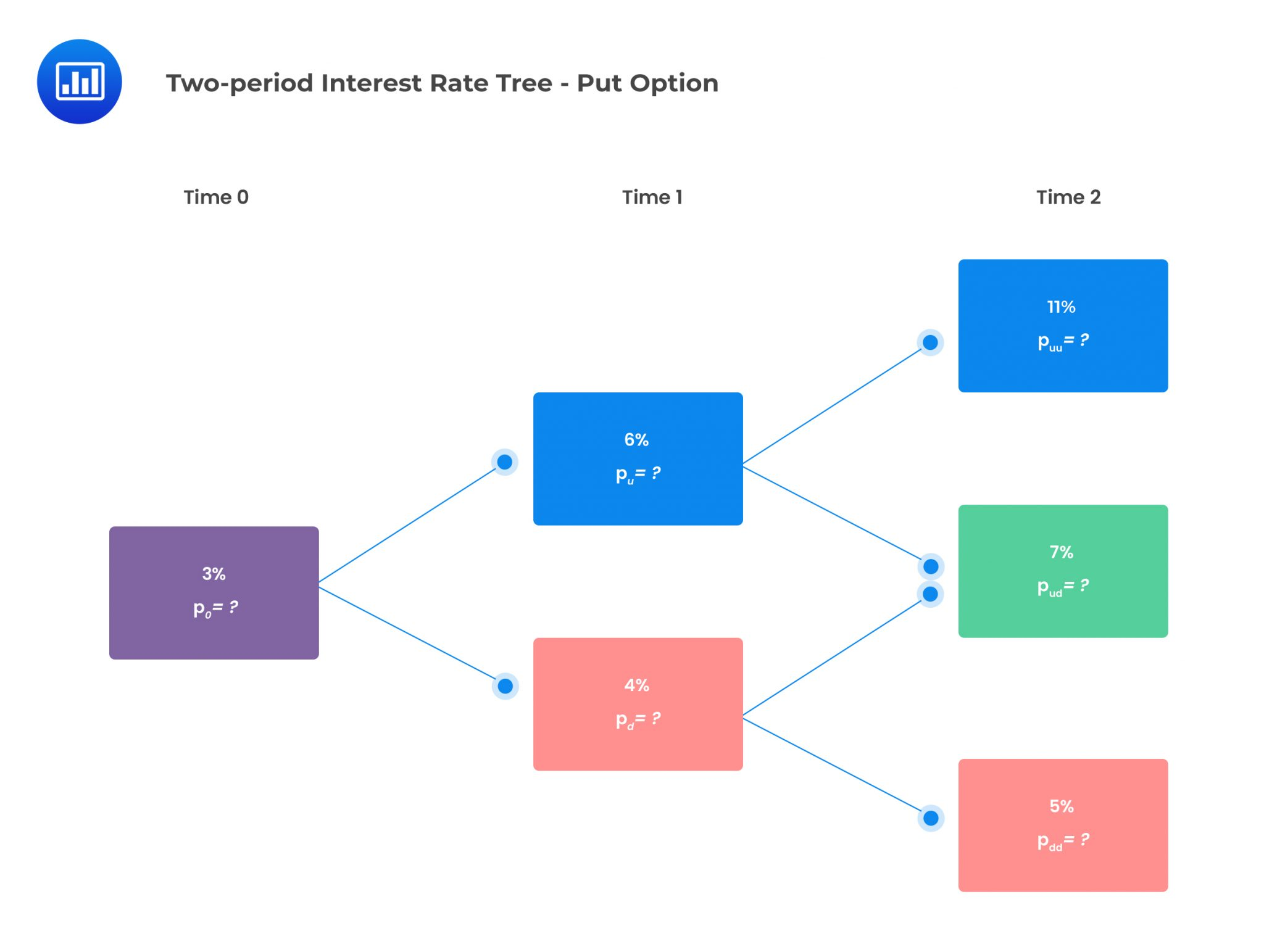# Valuation of an Interest Rate Option (2022 curriculum)

Interest rate options are options with an interest rate as the underlying. A call option on interest rates has a positive payoff when the current spot rate is greater than the exercise rate.

$$\text{Call option payoff}=\text{Notional Amount}\times[\text{Max}(\text{Current spot rate}-\text{Exercise rate, 0})]$$

On the other hand, a put option on interest rates has a positive payoff when the current spot rate is less than the exercise rate.

$$\text{Put payoff}=\text{Notional Amount}\times[\text{Max}(\text{Exercise rate}-\text{Current spot rate},0)]$$

We can apply the binomial model to value such interest rate options.

### Example: Calculating the value of an interest rate European option

Consider a two-year European-style call option with a one-year spot rate compounded annually as the underlying. The exercise rate is 6%. The two-period interest rate tree is given below:Suppose that the notional principal of each option is 500,000, and the risk-neutral probability of an up jump is 0.5. The value of the European call option can be determined as follows: #### Payoffs at time 2 \begin{align*}\text{c}_{\text{uu}}&=\text{Max}(0,\text{S}_{0}\text{u}^{2}-\text{K})\\&=\text{Max}(0,0.11-0.06)\\&=0.05\end{align*} \begin{align*}\text{c}_{\text{ud}}&=\text{Max}(0,\text{S}_{0}\text{ud}-\text{K})\\&=\text{Max}(0,0.07-0.06)\\&=0.01\end{align*} \begin{align*}\text{c}_{\text{dd}}&=\text{Max}(0,\text{S}_{0}\text{d}^{2}-\text{K})\\&=\text{Max}(0,0.05-0.06)\\&=0\end{align*} #### Value of call at time 1 \begin{align*}c_{u}&=PV_{1,2}[qc_{uu}+(1+q)c_{ud}]\\&=\frac{1}{1.06}[0.5\times0.05+(1-0.5)\times0.01]\\&=0.028302\end{align*} \begin{align*}c_{d}&=PV_{1,2}[qc_{ud}+(1-q)c_{dd}]\\&=\frac{1}{1.04}[0.5\times0.01+(1-0.5)\times0]\\&=0.004808\end{align*} #### Value of call at time 0 \begin{align*}c_{0}&=PV_{0,1}[qc_{u}+(1-q)c_{d}]\\&=\frac{1}{1.03}[0.5\times0.028302+(1-0.5)\times0.004808]\\&=0.01607\end{align*} The call value at time 0 is then obtained by multiplying with the notional amount: $$\text{Call value}=0.01607\times500,000=8,035$$ ## Question Consider a two-year European-style put option with the annually compounded one-year spot interest rate as the underlying. The exercise rate is 6%. The two-period interest rate tree is given below:Suppose that the notional principal of each option is500,000, and the risk-neutral (RN) probability of an up jump is 0.5. The value of the European put option is closest to:

1. $1,167 2.$5,835
3. \$8,035

### Solution

#### Put Payoffs at time 2

Similar to a call option, a put option will have three possible payoffs:

\begin{align*}p_{uu}&=Max(0,K-S_{0}u^{2})\\&=Max(0,0.06-0.11)\\&=0\end{align*}

\begin{align*}p_{ud}&=Max(0,K-S_{0}ud)\\&=Max(0,0.06-0.07)\\&=0\end{align*}

\begin{align*}p_{dd}&=Max(0,K-S_{0}dd)\\&=max(0,0.06-0.05)\\&=0.01\end{align*}

#### Value of put at time 1

\begin{align*}p_{u}&=PV_{1,2}[qp_{uu}+(1-q)p_{ud}]\\&=\frac{1}{1.06}[0.5\times0+(1-0.5)\times0]\\&=0\end{align*}

\begin{align*}p_{d}&=PV_{1,2}[qp_{ud}+(1-q)c_{dd}]\\&=\frac{1}{1.04}[0.5\times0+(1-0.5)\times0.01]\\&=0.004808\end{align*}

#### Value of put at time 0

\begin{align*}p_{0}&=PV_{0,1}[qp_{u}+(1-q)p_{d}]\\&=\frac{1}{1.03}[0.5\times0+(1-0.5)\times0.004808]\\&=0.00233398\end{align*}

The put value at time 0 is then obtained by multiplying by the notional amount:

$$\text{put value}=0.002334\times500,000=1,167$$

Reading 38: Valuation of Contingent Claims

LOS 38 (d) calculate and interpret the value of an interest rate option using a two-period binomial model;

Shop CFA® Exam Prep

Offered by AnalystPrepLevel I
Level II
Level III
All Three Levels
Featured Shop FRM® Exam PrepFRM Part I
FRM Part II
FRM Part I & Part II
Learn with Us

Subscribe to our newsletter and keep up with the latest and greatest tips for success
Shop Actuarial Exams PrepExam P (Probability)
Exam FM (Financial Mathematics)
Exams P & FMGMAT® Complete CourseDaniel Glyn
2021-03-24
I have finished my FRM1 thanks to AnalystPrep. And now using AnalystPrep for my FRM2 preparation. Professor Forjan is brilliant. He gives such good explanations and analogies. And more than anything makes learning fun. A big thank you to Analystprep and Professor Forjan. 5 stars all the way!michael walshe
2021-03-18
Professor James' videos are excellent for understanding the underlying theories behind financial engineering / financial analysis. The AnalystPrep videos were better than any of the others that I searched through on YouTube for providing a clear explanation of some concepts, such as Portfolio theory, CAPM, and Arbitrage Pricing theory. Watching these cleared up many of the unclarities I had in my head. Highly recommended.Nyka Smith
2021-02-18
Every concept is very well explained by Nilay Arun. kudos to you man!2021-02-13